# EU and US GDP by Region, Fourth Quarter 2019

Apr 28, 2020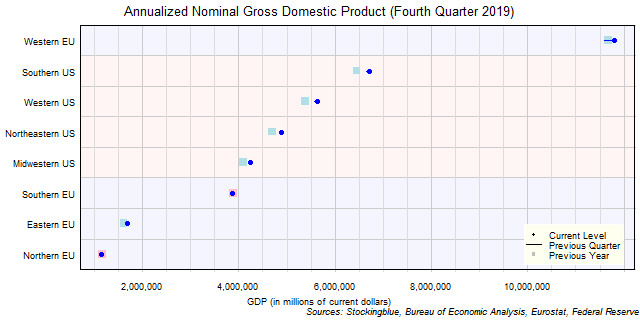The chart above shows the annualized nominal gross domestic product (GDP) in each EU and US region as of the fourth quarter of 2019 in millions of dollars, the change from the previous quarter, and the GDP one year prior.  The Western EU is the only EU region that has a larger GDP than any US region.

# EU GDP by Region, Fourth Quarter 2019

Apr 27, 2020The chart above shows the annualized nominal gross domestic product (GDP) in each EU region as of the fourth quarter of 2019 in millions of euros, the change from the previous quarter, and the GDP one year prior.  Every single region's economy grew both over the past quarter and the past year.

# US GDP by Region, Fourth Quarter 2019

Apr 24, 2020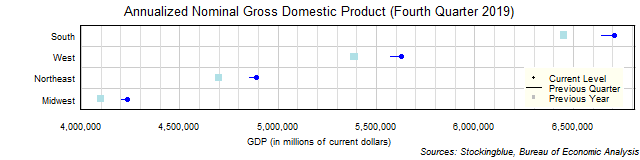The chart above shows the annualized nominal gross domestic product (GDP) in each US region as of the fourth quarter of 2019 in millions of dollars, the change from the previous quarter, and the GDP one year prior.  Every single region's economy grew both over the past quarter and the past year.

# EU and US GDP by State, Fourth Quarter 2019

Apr 23, 2020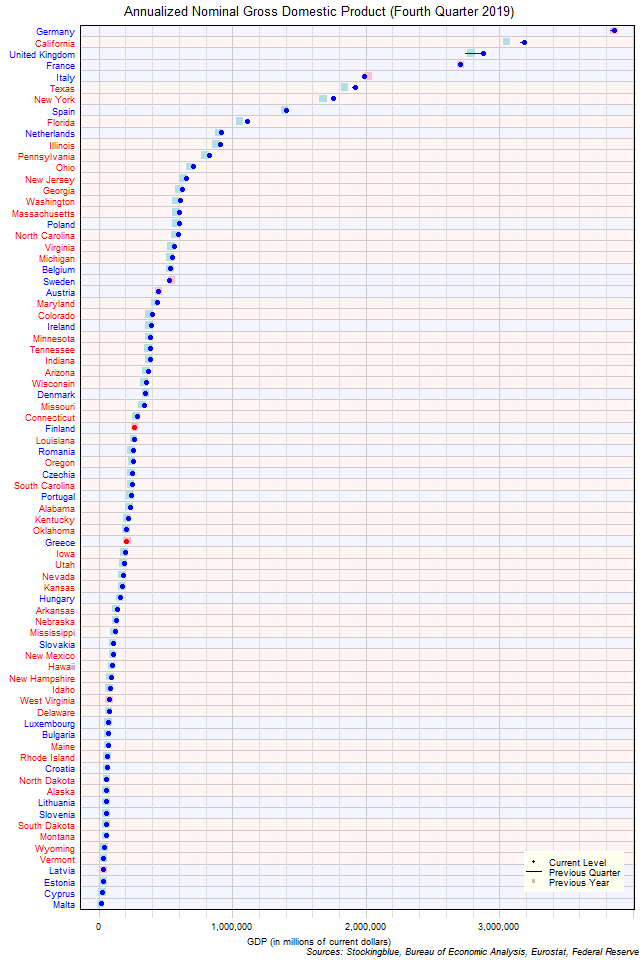The chart above shows the annualized nominal gross domestic product (GDP) in each EU and US state as of the fourth quarter of 2019 in millions of US dollars, the change from the previous quarter, and the GDP one year prior.  Only four states in both the EU and the US (three of which are from the EU) have an annualized GDP of over \$2 trillion.

# EU GDP by State, Fourth Quarter 2019

Apr 22, 2020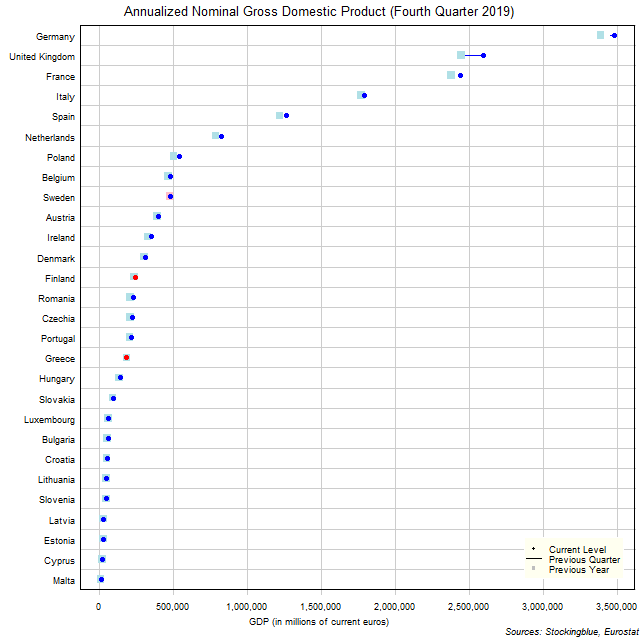The chart above shows the annualized nominal gross domestic product (GDP) in each EU state as of the fourth quarter of 2019 in millions of euros, the change from the previous quarter, and the GDP one year prior.  Sweden, Finland, and Greece are the only states to see a drop either from the previous quarter or the previous year.

# US GDP by State, Fourth Quarter 2019

Apr 21, 2020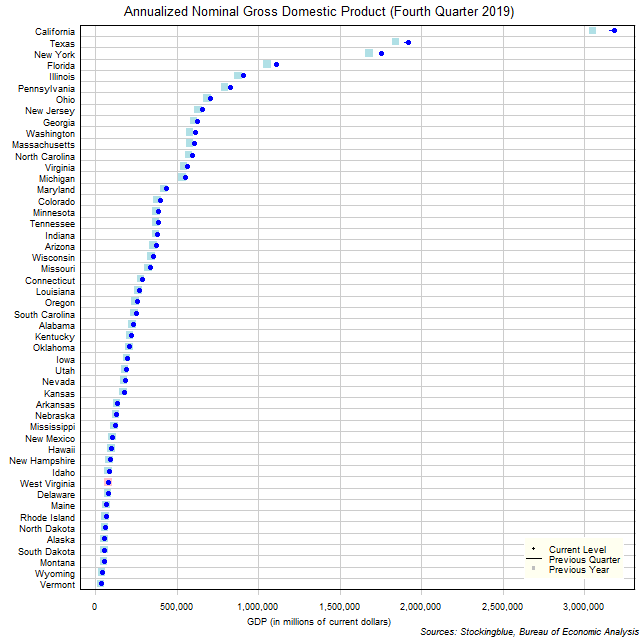The chart above shows the annualized nominal gross domestic product (GDP) in each US state as of the fourth quarter of 2019 in millions of dollars, the change from the previous quarter, and the GDP one year prior.  West Virginia was the only state to see a reduction in GDP over the previous year.

# Long-Term EU and US GDP Growth Rate by Region, Third Quarter 2019

Apr 10, 2020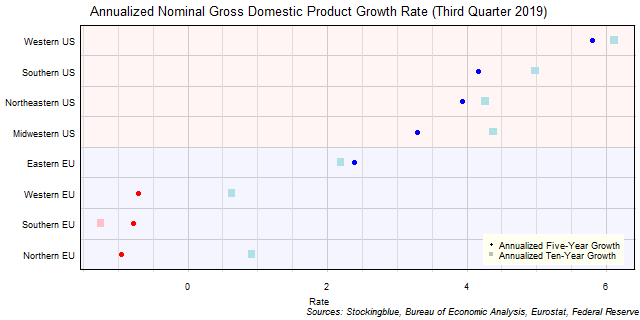The chart above shows the annualized nominal gross domestic product (GDP) growth rate when priced in US dollars in each EU and US region over the past five years as of the third quarter of 2019 and the growth over the past ten years.  All negative growth rates in the EU are attributed to currency rate fluctuations.

# Long-Term EU GDP Growth Rate by Region, Third Quarter 2019

Apr 9, 2020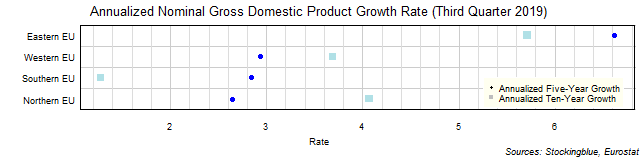The chart above shows the annualized nominal gross domestic product (GDP) growth rate in each EU region over the past five years as of the third quarter of 2019 and the growth rate over the past ten years.  The Western and Northern EU had a slower growth rate over the past five years than that had over the past ten years.

# Long-Term US GDP Growth Rate by Region, Third Quarter 2019

Apr 8, 2020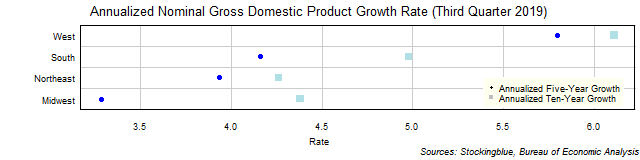The chart above shows the annualized nominal gross domestic product (GDP) growth rate in each US region over the past five years as of the third quarter of 2019 and the growth rate over the past ten years.  The West's growth rates outshine the other regions' rates.

# Long-Term EU and US GDP Growth Rate by State, Third Quarter 2019

Apr 3, 2020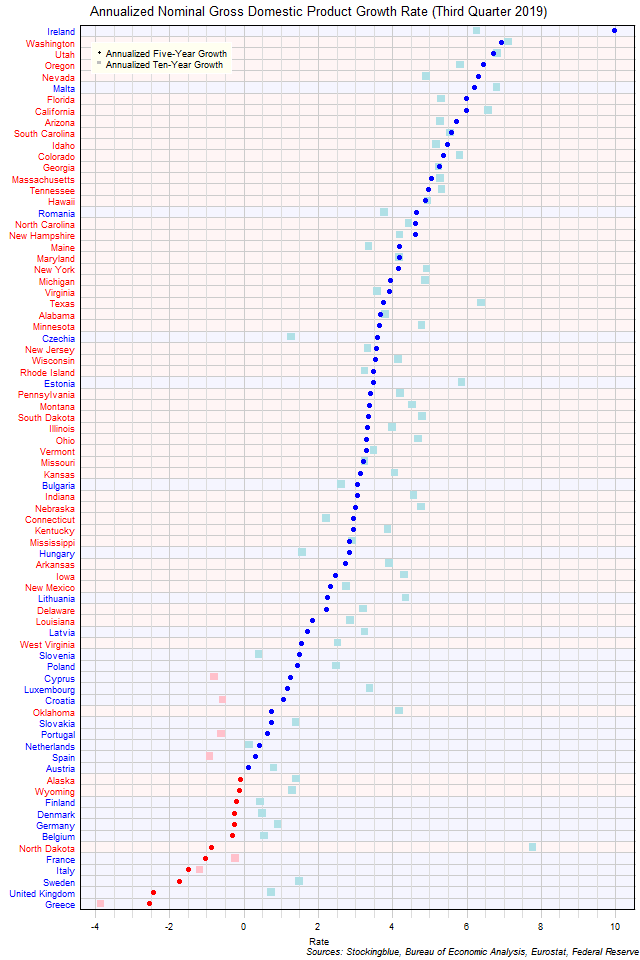The chart above shows the annualized nominal gross domestic product (GDP) growth rate when the GDP is priced in US dollars in each EU and US state over the past five years as of the third quarter of 2019 and the growth rate over the past ten years.  Only EU states saw a contraction both over the past five and ten years.